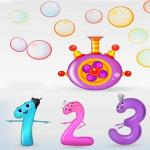Rate:Learn Math

Want kids to develop and improve math skills? Are you looking for a math game to practice skills for kids? Congratulations! Because I will introduce you to a math game which is extremely attractive and interesting for all ages, especially children. Challenge your intelligence and mathematical thinking with Learn Math game now!

In this game, your biggest task is to find the right answer for each calculation. Calculations are displayed on the screen such as addition, subtraction, multiplication, division, and decimal. After each calculation occurs, you need to calculate carefully and accurately to give the answer: True or False. You just need to press the 2 icons on the screen.

Orange to give the right answer, blue is the wrong answer. In this game at kids games friv, you will not have to suffer the stress of time limits. So you don’t have to hurry to give the answer, instead you have to calculate carefully and give the correct answer. Questions will come up with random calculations, so you have to focus on making the correct decisions. Try your best to win the highest score in the game.

Especially, when playing this game, you will have the opportunity to train and stimulate the ability to calculate in a short time. Who can correctly answer all the quizzes in this game? It could be you! Do you want to play many related games? Check out Math For Kids at http://friv3play.net/!

How to play?

Play the game by using the mouse on the computer.

Rating: 5 out of 5 based on 1 user ratings

Learn Math GamePlay:

Want kids to develop and improve math skills? Are you looking for a math game to practice skills for kids? Congratulations! Because I will introduce you to a math game which is extremely attractive and interesting for all ages, especially children. Challenge your intelligence and mathematical thinking with Learn Math game now!

In this game, your biggest task is to find the right answer for each calculation. Calculations are displayed on the screen such as addition, subtraction, multiplication, division, and decimal. After each calculation occurs, you need to calculate carefully and accurately to give the answer: True or False. You just need to press the 2 icons on the screen.

Orange to give the right answer, blue is the wrong answer. In this game at kids games friv, you will not have to suffer the stress of time limits. So you don’t have to hurry to give the answer, instead you have to calculate carefully and give the correct answer. Questions will come up with random calculations, so you have to focus on making the correct decisions. Try your best to win the highest score in the game.

Especially, when playing this game, you will have the opportunity to train and stimulate the ability to calculate in a short time. Who can correctly answer all the quizzes in this game? It could be you! Do you want to play many related games? Check out Math For Kids at http://friv3play.net/!

How to play?

Play the game by using the mouse on the computer.

Exit Fullscreen